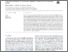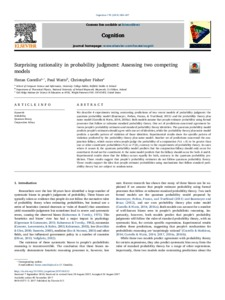# Surprising rationality in probability judgment: Assessing two competing models

Costello, Fintan and Watts, Paul and Fisher, Christopher (2018) Surprising rationality in probability judgment: Assessing two competing models. Cognition, 170. pp. 280-297. ISSN 0010-0277Previewmore...Add this article to your Mendeley library

## Abstract

We describe 4 experiments testing contrasting predictions of two recent models of probability judgment: the quantum probability model (Busemeyer, Pothos, Franco, & Trueblood, 2011) and the probability theory plus noise model (Costello & Watts, 2014, 2016a). Both models assume that people estimate probability using formal processes that follow or subsume standard probability theory. One set of predictions concerned agreement between people’s probability estimates and standard probability theory identities. The quantum probability model predicts people’s estimates should agree with one set of identities, while the probability theory plus noise model predicts a specific pattern of violation of those identities. Experimental results show the specific pattern of violation predicted by the probability theory plus noise model. Another set of predictions concerned the conjunction fallacy, which occurs when people judge the probability of a conjunction P ( ) A B ∧ to be greater than one or other constituent probabilities P (A) or P ( ) B , contrary to the requirements of probability theory. In cases where A causes B, the quantum probability model predicts that the conjunction fallacy should only occur for constituent B and not for constituent A: the noise model predicts that the fallacy should occur for both A and B. Experimental results show that the fallacy occurs equally for both, contrary to the quantum probability prediction. These results suggest that people’s probability estimates do not follow quantum probability theory. These results support the idea that people estimate probabilities using mechanisms that follow standard probability theory but are subject to random noise

Item Type: Article Probability; Quantum theory; Conjunction fallacy; Faculty of Science and Engineering > Theoretical Physics 13097 https://doi.org/10.1016/j.cognition.2017.08.012 Paul Watts 23 Jun 2020 15:28 Cognition Elsevier YesItem control page